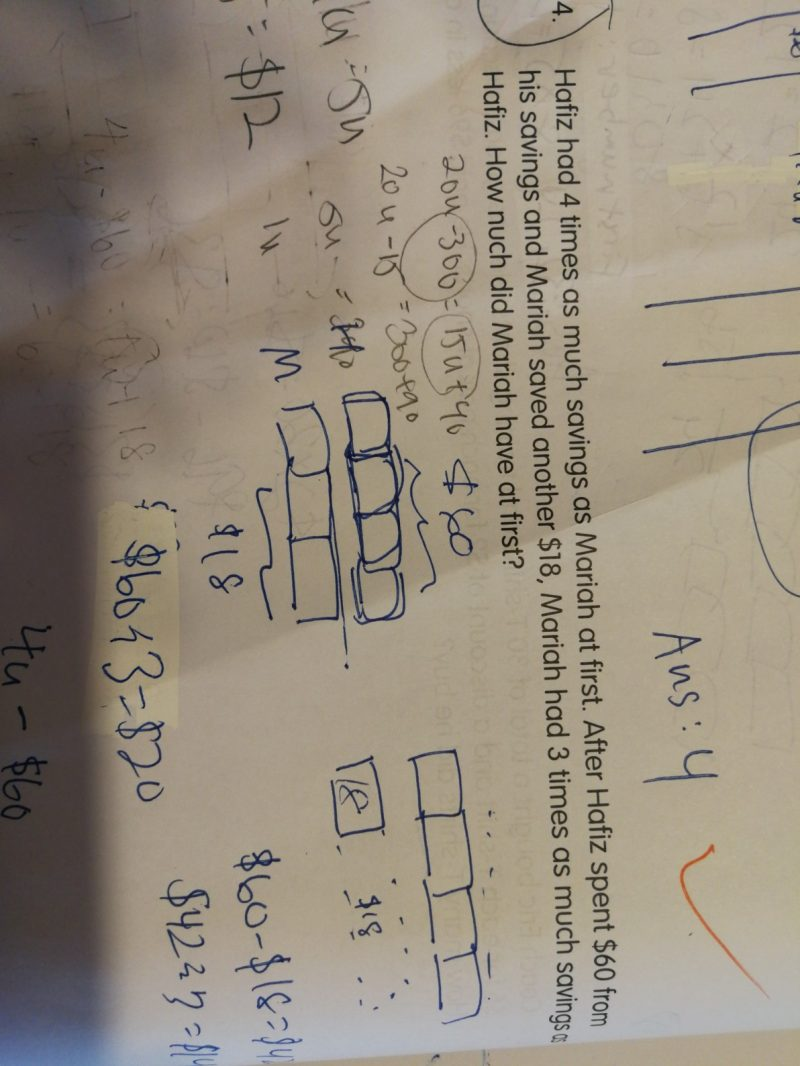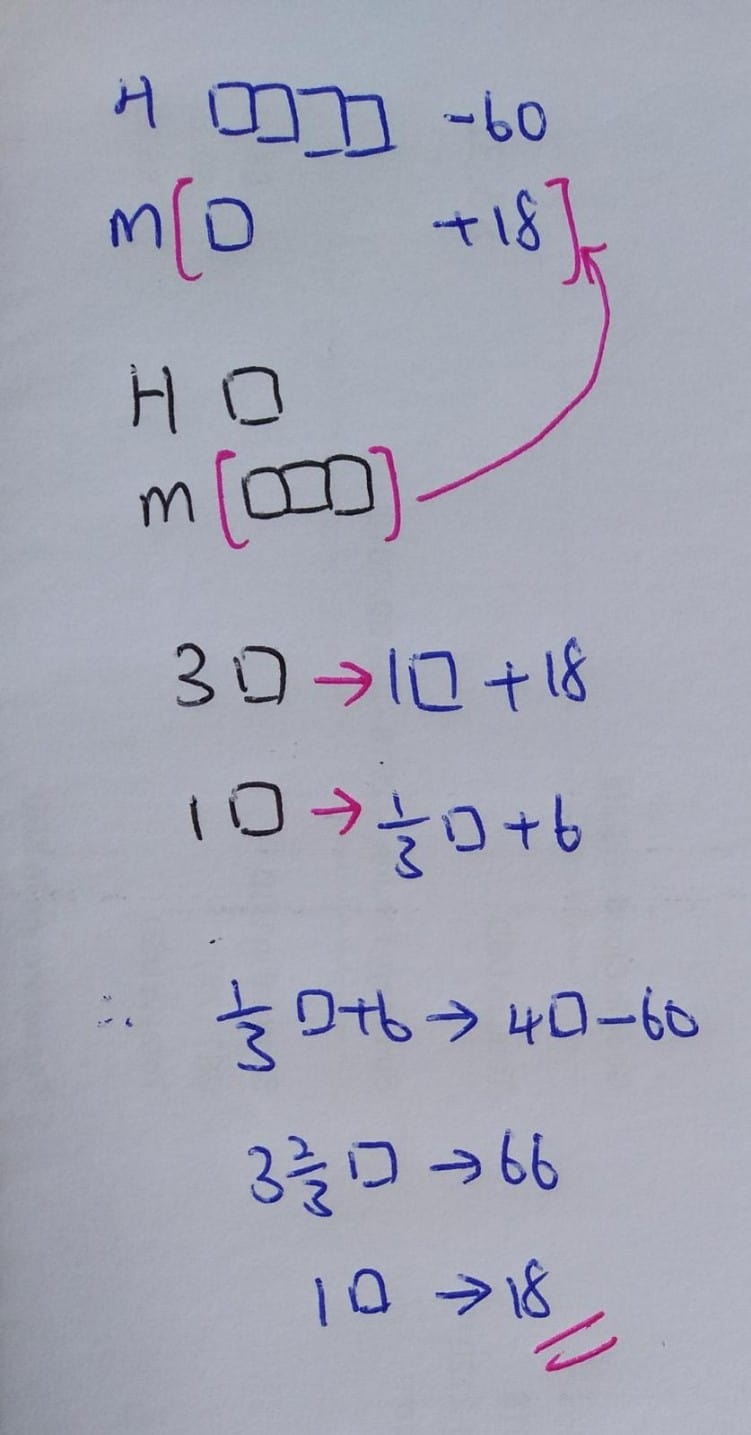# QuestionThanks0 Replies 0 Likes

At first,

H : M = 4 : 1 = 4u : u

After the changes,

(4u-60) : (u +18) = 1 : 3

=>

u +18 = 3 x (4u – 60)

u + 18 = 12u – 180

12u – u = 180 + 18

u = 18

M = u = 18

Hi

Can u explain why need the 3x is on Hafiz instead of Mariah?

Question stated Mariah had 3 times as much as Hafiz.

u +18 = 3 x (4u – 60)  – i dun understand this part.

Hi,

Mariah had 3 times as much as Hafiz; therefore,

M           =   3                               H

as

M= u + 18

H=4u-60

(u+18) = 3 (4u-60)

0 Replies 0 Likes